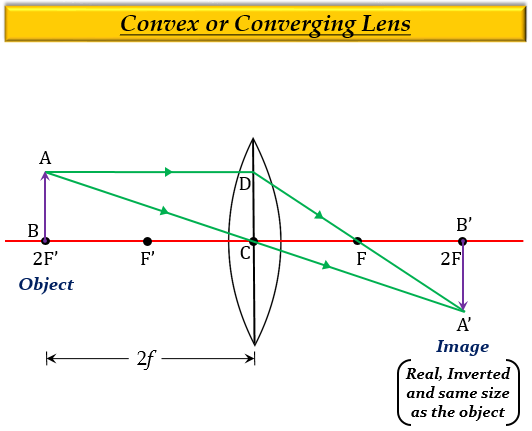# If the image formed by a convex lens is of the same size as that of the object, what is the position of the image with respect to the lens?

If the image formed by a convex lens is of the same size as that of the object, then the position of the image with respect to the lens is at a distance of $2f$ from the lens and behind it.

Explanation

When an object is at the focus $(2F')$ of a convex lens, which means the object is at a distance equal to twice the focal length, $2f$ of a convex lens, the image formed is real, inverted, same size as the object, and at a distance $2f$ on the other side of the lens.Updated on: 10-Oct-2022

261 Views